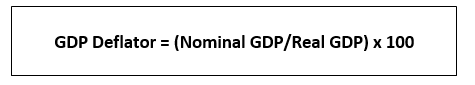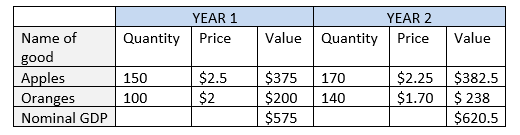# GDP Deflator

• Updated on

## What is GDP Deflator?

The GDP deflator is a measure of inflation defined over a specific period. It is the ratio of the value of goods and services produced in an economy in the current period to the value of these goods and services produced in the base year. The base year is the reference period starting from which price rise is calculated.

The GDP deflator is a price index which can be used to convert nominal GDP into real GDP. It is called a deflator as it is used to deflate the prices to the base year level. Generally, prices increase with time; thus, prices need to be deflated to achieve the real GDP.

Nominal GDP is the total value of all goods and services produced in a year. It is expressed in monetary terms. The price and quantity both belong to the current year. On the other hand, in real GDP, the quantity produced is from the current year while the prices are of the base year. Real GDP adjusts any changes in the prices while nominal GDP does not.

## Why is there a need for GDP deflator?

When the prices of goods and services increase over a year, then it is said that there has been inflation in prices. When the prices of goods and services become inflated, it gives a misleading picture of the production in the economy. This can give an inaccurate image of the income level in the economy.

Consider the situation where prices increase in the economy over a year, but the quantity produced in that year does not change. Going by the definition of nominal GDP, the value of goods and services (quantity * price) produced in that year would change. Thus, nominal GDP changes even when there is no change in the amount of goods and serviced produced.

Calculating the real GDP would show the actual picture. Real GDP includes prices of base year and quantity of current year. In the above example, when the quantity produced does not change, the real GDP remains the same. It does not give a bloated image of the production in the economy.

As nominal GDP rises without an actual rise in production, people expect their nominal income to increase as well. However, the real income in their hands remains the same. Therefore, to counterbalance inflation, an index is used so that the real price level in the economy can be realised.

## How is GDP deflator calculated?

The following formula gives the GDP Deflator:Consider the hypothetical case where an economy only produces apples and oranges. The prices of both apples and oranges change over a year, from Year 1 to Year 2, and so does their production. The change in prices and quantities can be summarised in the following table:Now for these prices and quantities, nominal GDP for Year 2 would be equal to the total value of goods and services in the economy. Nominal GDP in Year 2 is \$620.5 (= \$382.5 + \$238).

Now taking Year 1 as the base year, real GDP in Year 2 can be arrived at by multiplying the quantity in Year 2 with the prices in Year 1. Therefore, real GDP in Year 2 is \$705 {= (\$2.5 * 170) + (\$2 * 140)}.

Therefore, by the formula of GDP deflator, we get the following for Year 2:

GDP Deflator = (620.5 / 705) * 100 = 88.01

A GDP deflator of 88.01% means that the prices have fallen by 12% in Year 2 as compared to the base year, which, in this case, is Year 1. When the GDP deflator exceeds 100% it means that prices have increased.

The prices of both apples and oranges have fallen in Year 2. However, the nominal GDP has increased in the economy in Year 2, from \$575 to \$620.5. As prices decline, the quantity demanded of both the goods increases as stated by the Law of Demand. The effect of decreased prices is overshadowed by the impact of increased quantities, which explains why nominal GDP increased overall, even when the prices dropped.

## What is the difference between GDP deflator and other measures of inflation?

There are indexes other than the GDP deflator that can be used to adjust for inflation. One example of such an index is the Consumer Price Index. However, there is an underlying difference between assumptions of the two indexes. Consumer Price Index assumes that the quantity of goods produced in the economy remains the same, while GDP deflator does not include any such assumption.

In this sense, GDP deflator is a better measure of price inflation. It accounts for changes in the basket of goods produced as well as the changes in the prices of these goods. CPI fails to include the entire production in the economy.

Another commonly used index is the Wage Price Index (WPI). Like CPI, WPI also does not consider any changes in the basket of consumption. Also, WPI does not include the services sector in it. Because of these reasons, GDP deflator is preferred over WPI and CPI. However, CPI and WPI are published monthly because the GDP deflator can only be calculated with a lag. Therefore, CPI and WPI are used to track monthly changes in inflation.

##### Top ASX Listed Companies

Definition:
We use cookies to ensure that we give you the best experience on our website. If you continue to use this site we will assume that you are happy with it. OK